## Gene Density Ideograms

Since karyoploteR 1.8 it is possible to plot on the ideogram regions using the `data.plot="ideogram"`. In addtion to plotting on the ideograms, there’s the possibility of plotting something instead of the ideograms.

In this example we’ll see how to use the ideogram data panel to create a karyoplot where ideograms are replaced by the gene density levels. We’ll use the gene position information from the TxDb.Hsapiens.UCSC.hg19.knownGene Bioconductor package.

We’ll start by using the `genes` function to create a GRanges object with all genes.

``````library(TxDb.Hsapiens.UCSC.hg19.knownGene)
txdb <- TxDb.Hsapiens.UCSC.hg19.knownGene
all.genes <- genes(txdb)
``````
``````## GRanges object with 6 ranges and 1 metadata column:
##             seqnames              ranges strand |     gene_id
##                <Rle>           <IRanges>  <Rle> | <character>
##           1    chr19   58858172-58874214      - |           1
##          10     chr8   18248755-18258723      + |          10
##         100    chr20   43248163-43280376      - |         100
##        1000    chr18   25530930-25757445      - |        1000
##       10000     chr1 243651535-244006886      - |       10000
##   100008586     chrX   49217763-49233491      + |   100008586
##   -------
##   seqinfo: 93 sequences (1 circular) from hg19 genome
``````

And then, use the `kpPlotDensity` function to plot the gene density over the genome. We’ll start with 1 chromosome to get a better view and then we’ll move to the whole genome.

``````library(karyoploteR)

kp <- plotKaryotype(plot.type=1, chromosomes="chr1")
kp <- kpPlotDensity(kp, all.genes)
``````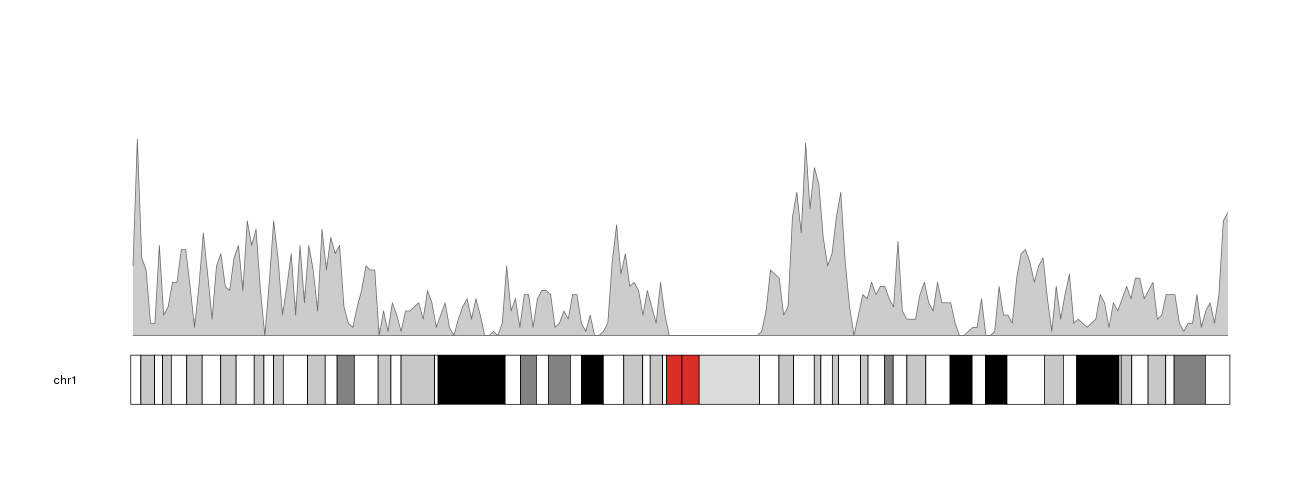We can see the plot density computed with the default window size, 1 megabse. Changing the window size to 500 kilobases we’ll get a less smoothed version of the data. We can also enlarge the chromosome name changing the `cex` parameter (character expansion) in `plotKaryotype`.

``````kp <- plotKaryotype(plot.type=1, chromosomes="chr1", cex=1.6)
kpPlotDensity(kp, all.genes, window.size = 0.5e6)
``````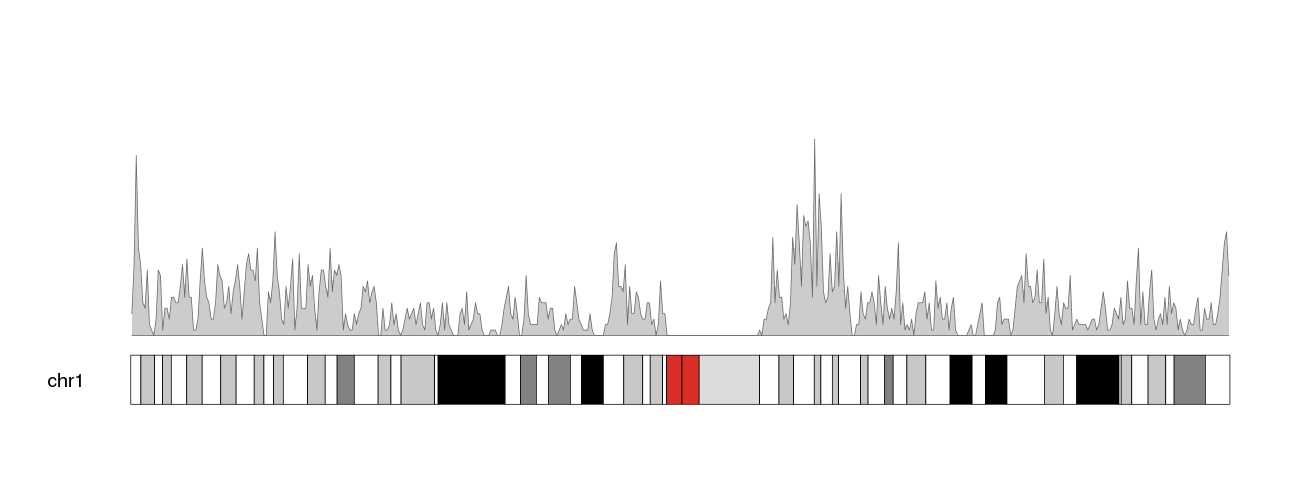Now, to move this data into the ideogram we simply need to specify that as the data panel with `data.panel="ideogram"`. We’ll get the exact same representation but on the ideogram.

``````kp <- plotKaryotype(plot.type=1, chromosomes="chr1", cex=1.6)
kpPlotDensity(kp, all.genes, window.size = 0.5e6, data.panel="ideogram")
``````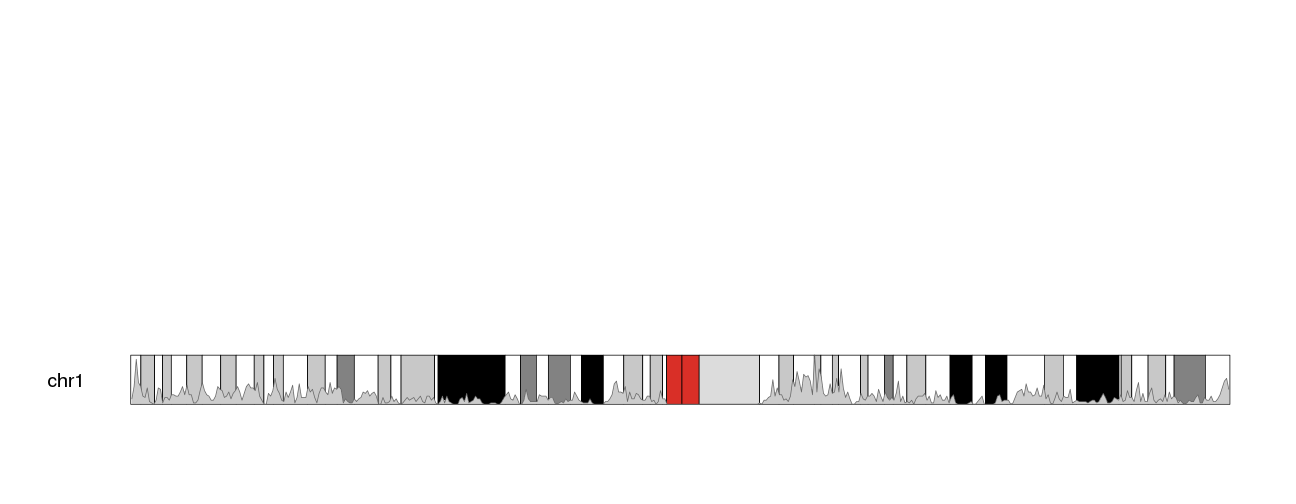The problem now is that the whole standard data panel is empty. If we do not want to use it to plot anything else, we can use one of the ideogram only plot types: `plot.type=6` for chromosomes arranged in a stack or `plot.type=7` for all chomosomes in a single line. With these plot types, standard data panels are removed and only the ideogram is available.

``````kp <- plotKaryotype(plot.type=6, chromosomes="chr1", cex=1.6)
kpPlotDensity(kp, all.genes, window.size = 0.5e6, data.panel="ideogram")
``````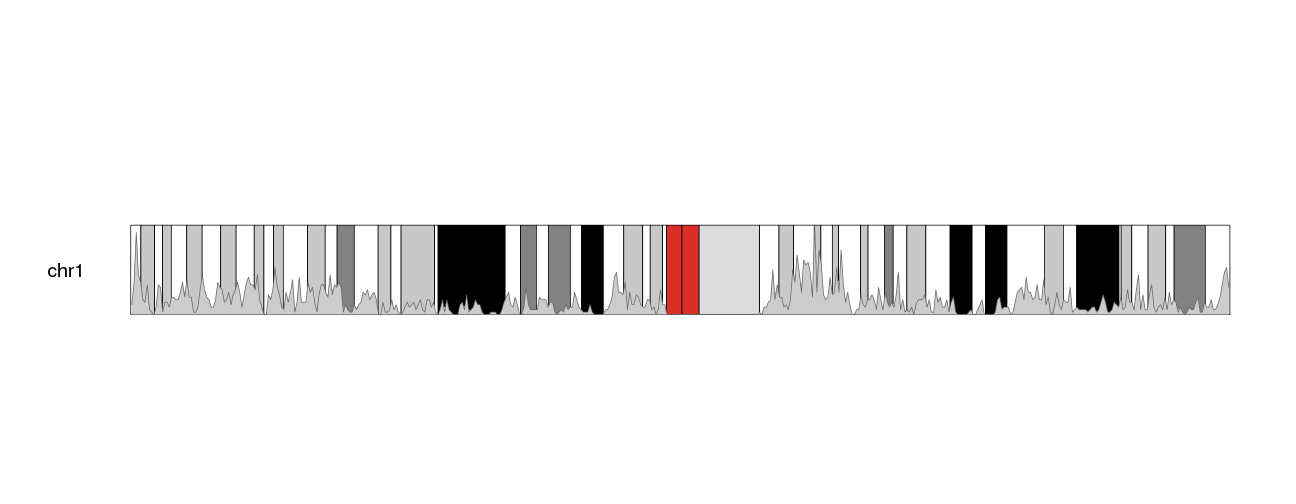The other problem we have is that its difficult to see the density data. We’ll change the color (bot the border and the shaded area) to make it more apparent.

``````kp <- plotKaryotype(plot.type=6, chromosomes="chr1", cex=1.6)
kpPlotDensity(kp, all.genes, window.size = 0.5e6, data.panel="ideogram", col="#3388FF", border="#3388FF")
``````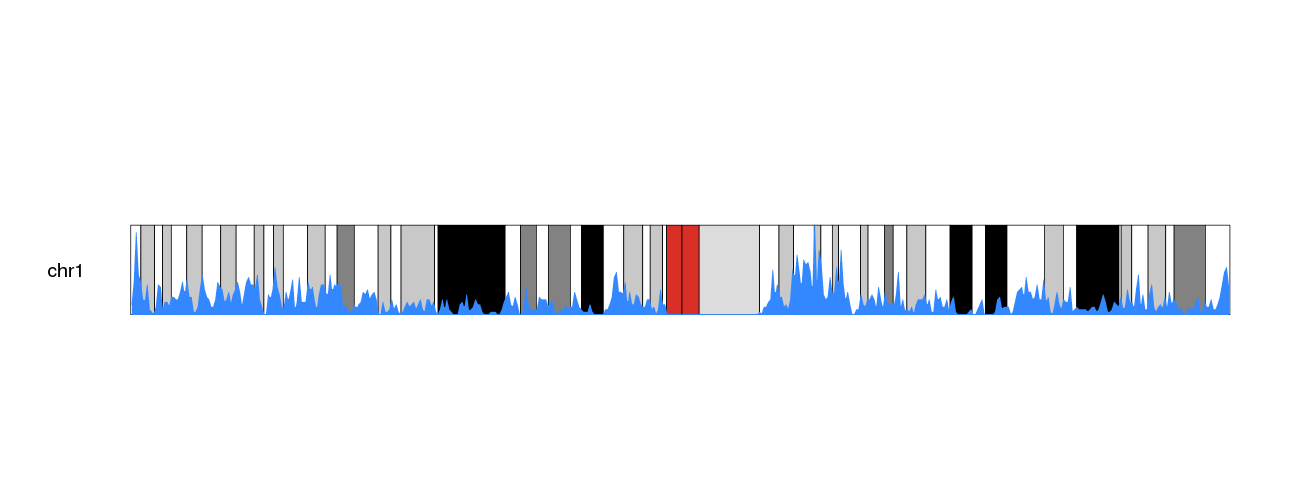We can then use the trick of duplicating the plot and inverting one of them with an r0 smaller than r1 to get a more interesting.

``````kp <- plotKaryotype(plot.type=6, chromosomes="chr1", cex=1.6)
kpPlotDensity(kp, all.genes, window.size = 0.5e6, data.panel="ideogram", col="#3388FF", border="#3388FF", r0=0.5, r1=1)
kpPlotDensity(kp, all.genes, window.size = 0.5e6, data.panel="ideogram", col="#3388FF", border="#3388FF", r0=0.5, r1=0)
``````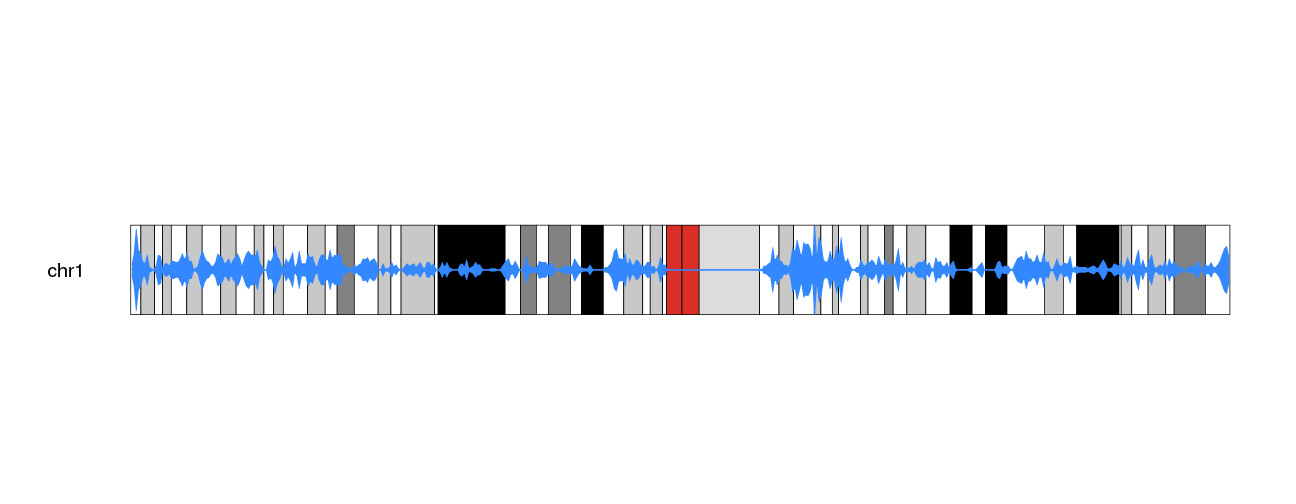And to the the plot density popping out more from the G-banding colors, we can dim the cytobands intensity. To do that we could get the color table with `getCytobandColors()` and modify them before calling `plotKaryotype()` or we could simply plot a white semi-transparent rectangle covering the whole ideograms. We can plot those rectamgles with `kpDataBackground` and specify a semitransparent color with the 2 last digits of the color definition in hexadecimal (i.e. “#FF0000” is red and “#FF0000AA”, is a semi-transparent red).

``````kp <- plotKaryotype(plot.type=6, chromosomes="chr1", cex=1.6)
kpDataBackground(kp, color = "#FFFFFFAA")
kpPlotDensity(kp, all.genes, window.size = 0.5e6, data.panel="ideogram", col="#3388FF", border="#3388FF", r0=0.5, r1=1)
kpPlotDensity(kp, all.genes, window.size = 0.5e6, data.panel="ideogram", col="#3388FF", border="#3388FF", r0=0.5, r1=0)
``````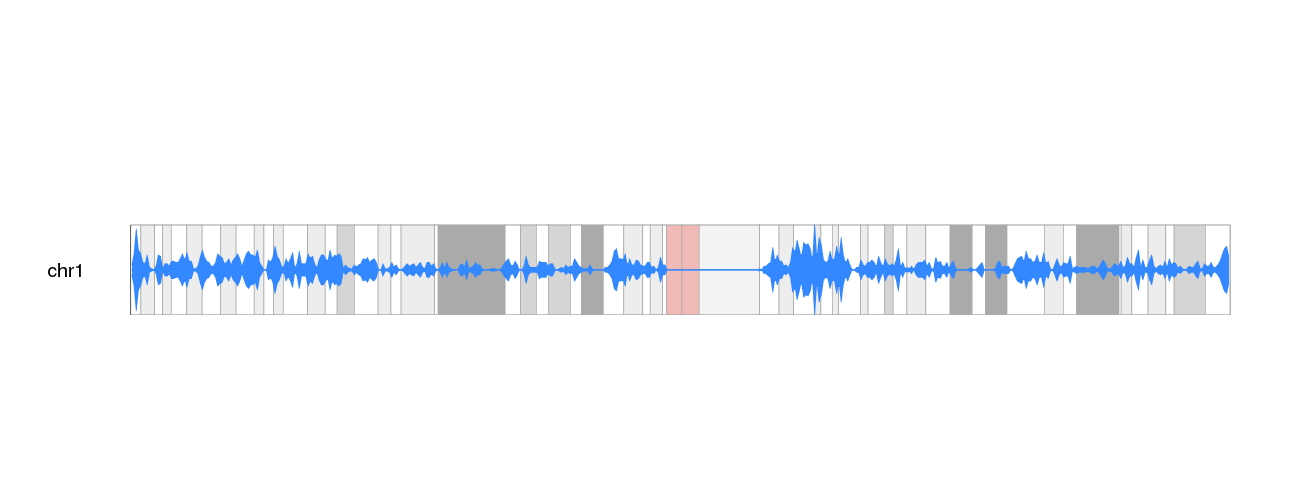We can now add a main title, and show all chromosomes to get the final plot of gene density on the ideograms.

``````kp <- plotKaryotype(plot.type=6, main="Gene Density",  cex=1.8)
kpDataBackground(kp, color = "#FFFFFFAA")
kp <- kpPlotDensity(kp, all.genes, window.size = 0.5e6, data.panel="ideogram", col="#3388FF", border="#3388FF", r0=0.5, r1=1)
kp <- kpPlotDensity(kp, all.genes, window.size = 0.5e6, data.panel="ideogram", col="#3388FF", border="#3388FF", r0=0.5, r1=0)
``````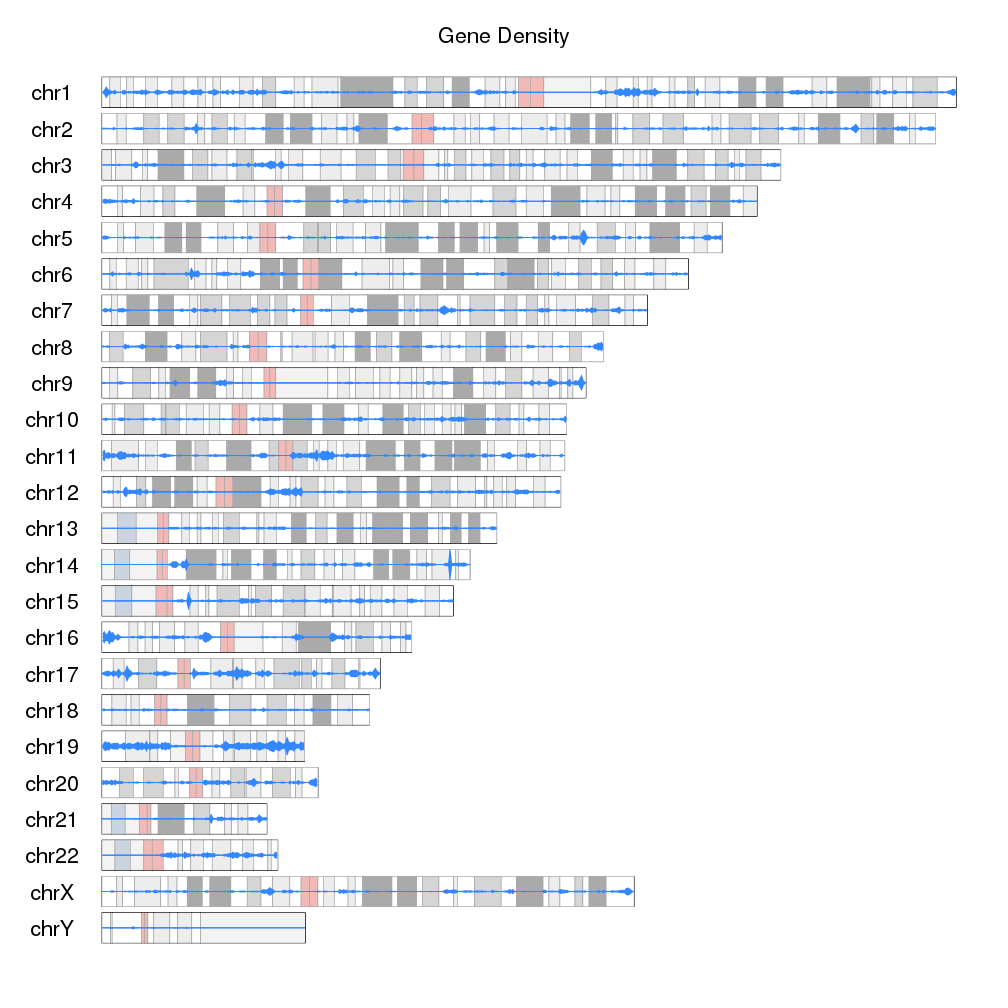If we want to go farther, we can actually replace the ideograms with the gene density data. To do that we’ll set `ideogram.plotter` to NULL in the call to `plotKaryotype` and plot the densities as we have been doing.

``````kp <- plotKaryotype(plot.type=6, main="Gene Density", ideogram.plotter = NULL, cex=1.8)
kp <- kpPlotDensity(kp, all.genes, window.size = 0.5e6, data.panel="ideogram", col="#3388FF", border="#3388FF", r0=0.5, r1=1)
kp <- kpPlotDensity(kp, all.genes, window.size = 0.5e6, data.panel="ideogram", col="#3388FF", border="#3388FF", r0=0.5, r1=0)
``````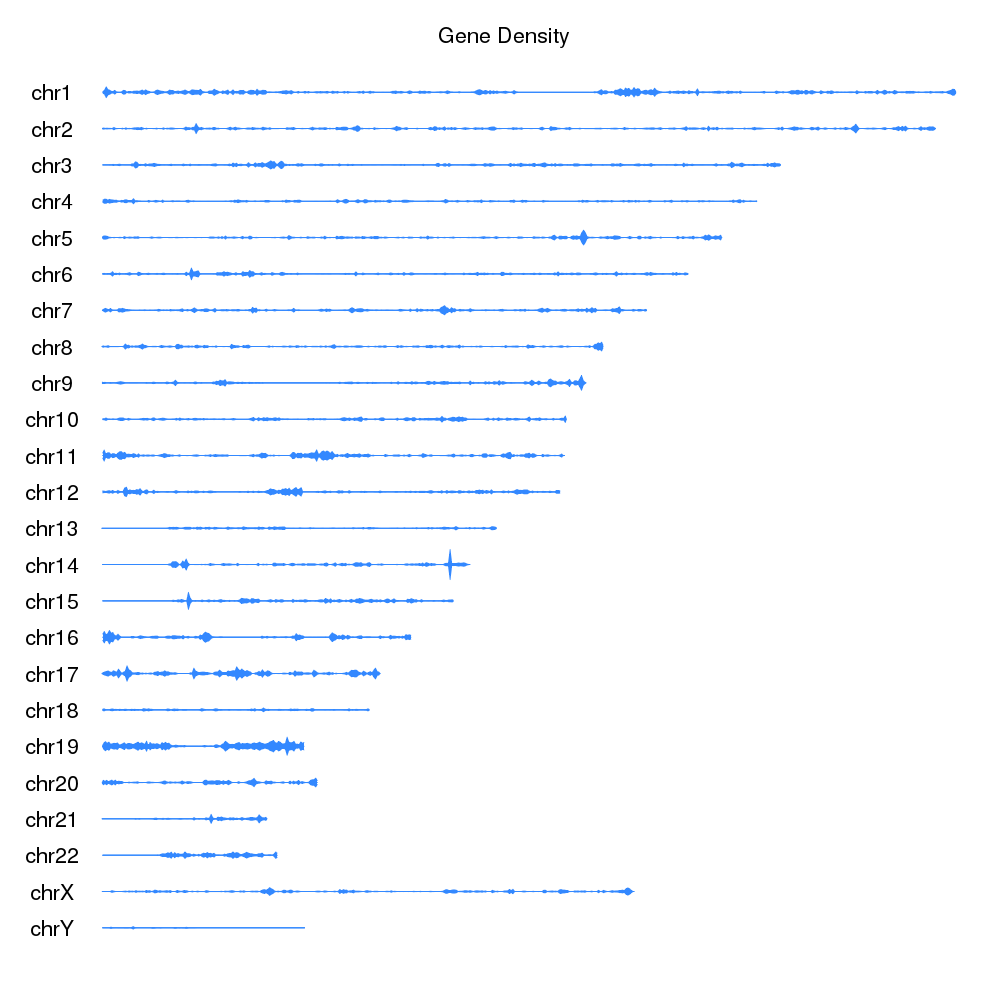We can use `data.panel="ideogram"` to use the ideogram as any other data.panel in karyoploteR and all plotting functions work on ideograms in the same way as they do in standard data panels.